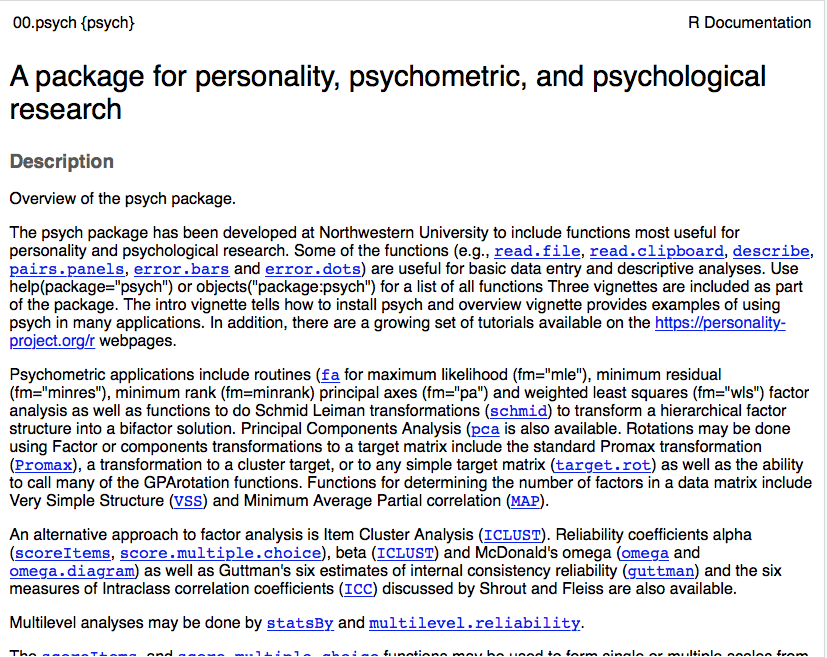## Introduction to R

The first step is to download and install R, which is freely available and can be accessed here. You can choose any of the “mirrors” you want, but it is best to use the one that is closest to you geographically (i.e., the one at Oregon State University).

The second step is to download and install Rstudio, which is a very nice integrated development environment (IDE) for R. In a nutshell, it makes working with R much easier. You will want to install the Rstudio Desktop version that is appropriate for your operating system. After you have installed R and Rstudio, you can procede to the next step.

### Getting started with R

R is a programming language that was developed to help researchers analyze quantitative data (e.g., do statistics). As such, R can be used for conducting both simple mathematical functions and complex statistical analyses. See below for some of the simple things that you can do with R. Note that any code directly preceded with a “#” is ignored by R.

``````# addition
1+2``````
``##  3``
``````#multiplication
5*4``````
``##  20``
``````#division
18/32``````
``##  0.5625``
``````#power calculations
5^2``````
``##  25``
``````#Square root
sqrt(25)``````
``##  5``

In short, you can use R as a calculator if you wish. ### Assigning variables We can also save values (or other objects) by assigning them to an arbitrary variable. We do this using “<-”.

``VarName1 <- 5^2``

Once we have saved a value (or other object) we can use it in later in various ways.

``print(VarName1) #display the value using the "print" function``
``##  25``
``VarName1 - 5``
``##  20``

R comes loaded with a large number of helpful packages and datasets. To access these datasets, we use the function library() which takes a package name as an argument.

``library("psych")``

If you haven’t installed the package “psych”, then you will need to install it, which you can do using the function install.packages(), which takes the name of the package you want to install as an argument.

``install.packages("psych")``

After installing the package, we can then load it. Note that we do NOT have to install packages each time we use R (we only have to do that once). We do, however, have to load the package each time we use R.

``library("psych")``

If we want to get details regarding the use of a particular package, we can use the help() function, which will open the documentation for the chosen package.

``help(psych)``### Playing with some data in R

R comes with a number of datasets pre-installed. Later on, we will load our own datasets, but for now, lets play with one that comes with R, called “mtcars”. First, lets see what kind of data mtcars comprises. We can do this using the help() function.

``help(mtcars)``